# 写在前面

Kipf 与 Welling 16 年发表的「Variational Graph Auto-Encoders」提出了基于图的（变分）自编码器 Variational Graph Auto-Encoder（VGAE），自此开始，图自编码器凭借其简洁的 encoder-decoder 结构和高效的 encode 能力，在很多领域都派上了用场。

Thomas N. Kipf大佬不仅是这篇VGAE的作者，而且还是大名鼎鼎模型GCN的作者。二作是CIFAR10实验室的Max Welling，他是GCNVAE的作者之一，Kipf是Max Welling的博士生。

# 一、引入图神经网络

 样本    特征x                标签y
31336: x=(0,1,0,0,0...0), y=Neural_Networks
10611: x=(0,0,1,0,1...0), y=Rule_Learning
...
12135: x=(0,0,0,0,0...0), y= Reinforcement_Learning

• 方法1：直接对P(y|x)建模（传统监督学习），模型性能一般
• 方法2：先基于图结构的图神经网络模型（如GCN）提取更好的表征(x->z)，再进行下游任务建模(P(y|z))，模型性能较好（这种方法就是图神经网络）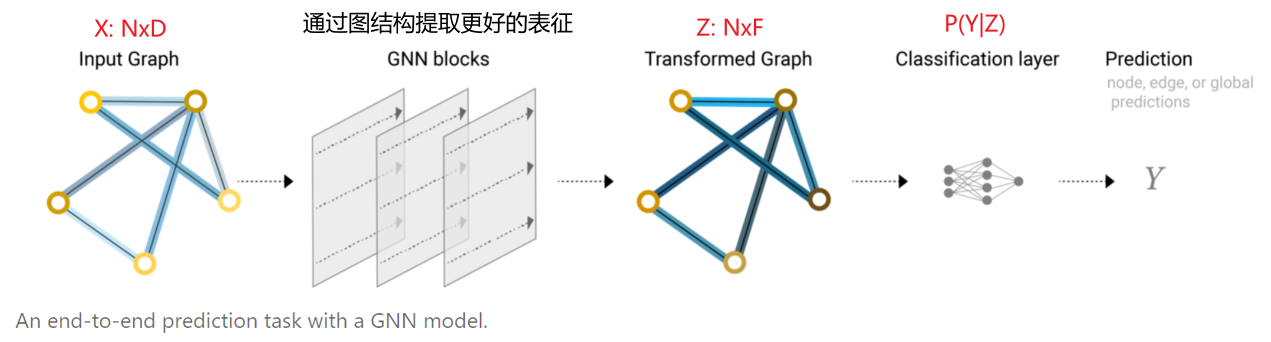• 类型1：监督学习或半监督学习是通过$A,x,y$来学习表征$z$
• 类型2：当图上没有标签$y$时，可以通过无监督学习的方式来学习$z$，即只通过$A,x$学习$z$

• GAE：图自编码器 Graph Auto-Encoder（主要用于压缩$A,x\rightarrow z$）
• VGAE：变分图自编码器 Variational Graph Auto-Encoder（主要用于图的生成$z\rightarrow A$）

# 二、GAE 图自编码器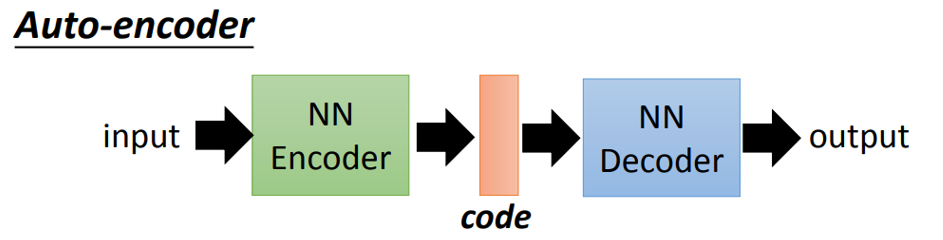GAE是GCN在Auto-Encoders的应用，非常容易理解，隐变量Z就是图上的N个节点经过GCN后的N*F维特征，Encoder就是两层GCN, Decoder就是向量点积。可以将隐变量Z理解为某种意义上图的节点的相似度，通过向量点积得到的两个图节点的相似度越大，则两个节点之间存在边的概率越大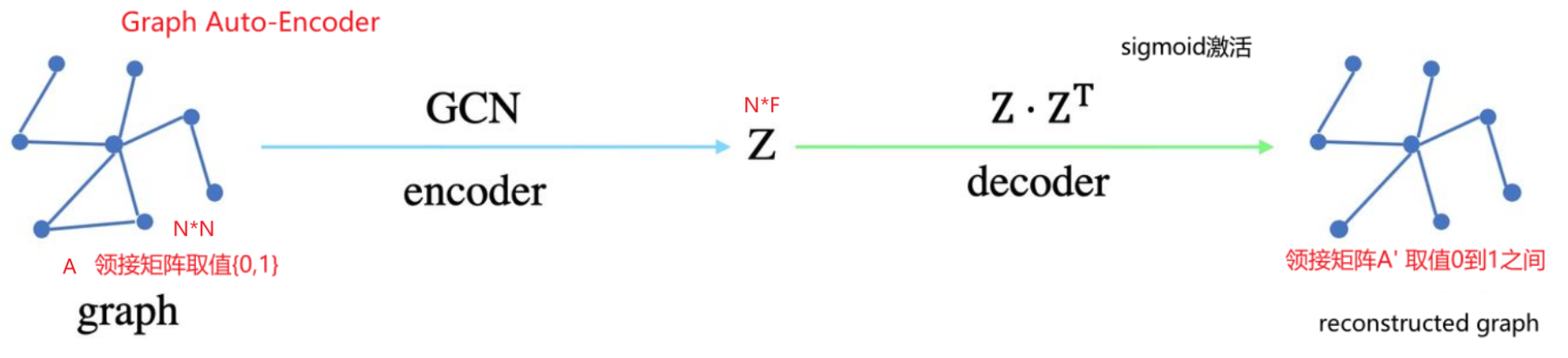• GAE使用GCN作为encoder来得到节点的latent representations
• $Z=GCN(X,A)=\hat{A}ReLU(\hat{A}XW_0)W_1$， 其中$\hat{A}={D}^{-\frac{1}{2}} {A} {D}^{-\frac{1}{2}}$，注意VGAE论文中的$A$已经加上了$I$
• 原论文的描述：We introduce an adjacency matrix A of G (we assume diagonal elements set to 1, i.e. every node is connected to itself) and its degree matrix D.
• GAE使用inner-product作为decoder来reconstruct原始的图
• $A’=\sigma(ZZ^T)=sigmoid(ZZ^T)$

GAE是如何训练，它的loss函数是什么？

$$\mathcal{Loss(A, A’)}=-\frac{1}{N} \sum y \log {y’}+(1-y) \log (1-{y’})$$

Networks》https://arxiv.org/pdf/1901.00596.pdf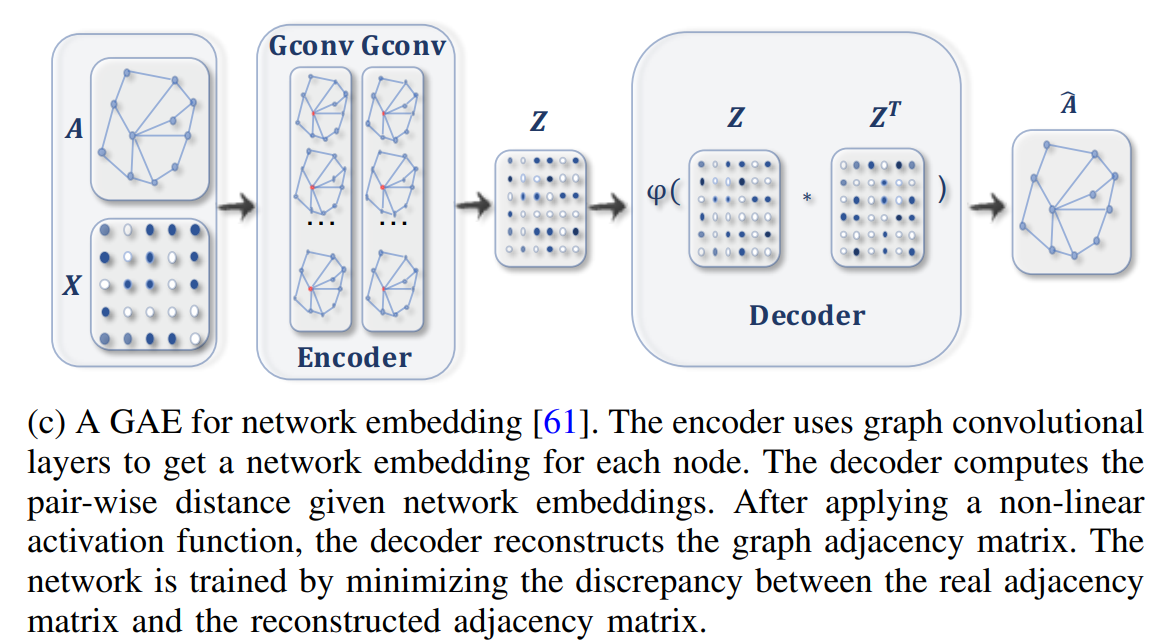# 三、VGAE 图变分自编码器

• VAE训练阶段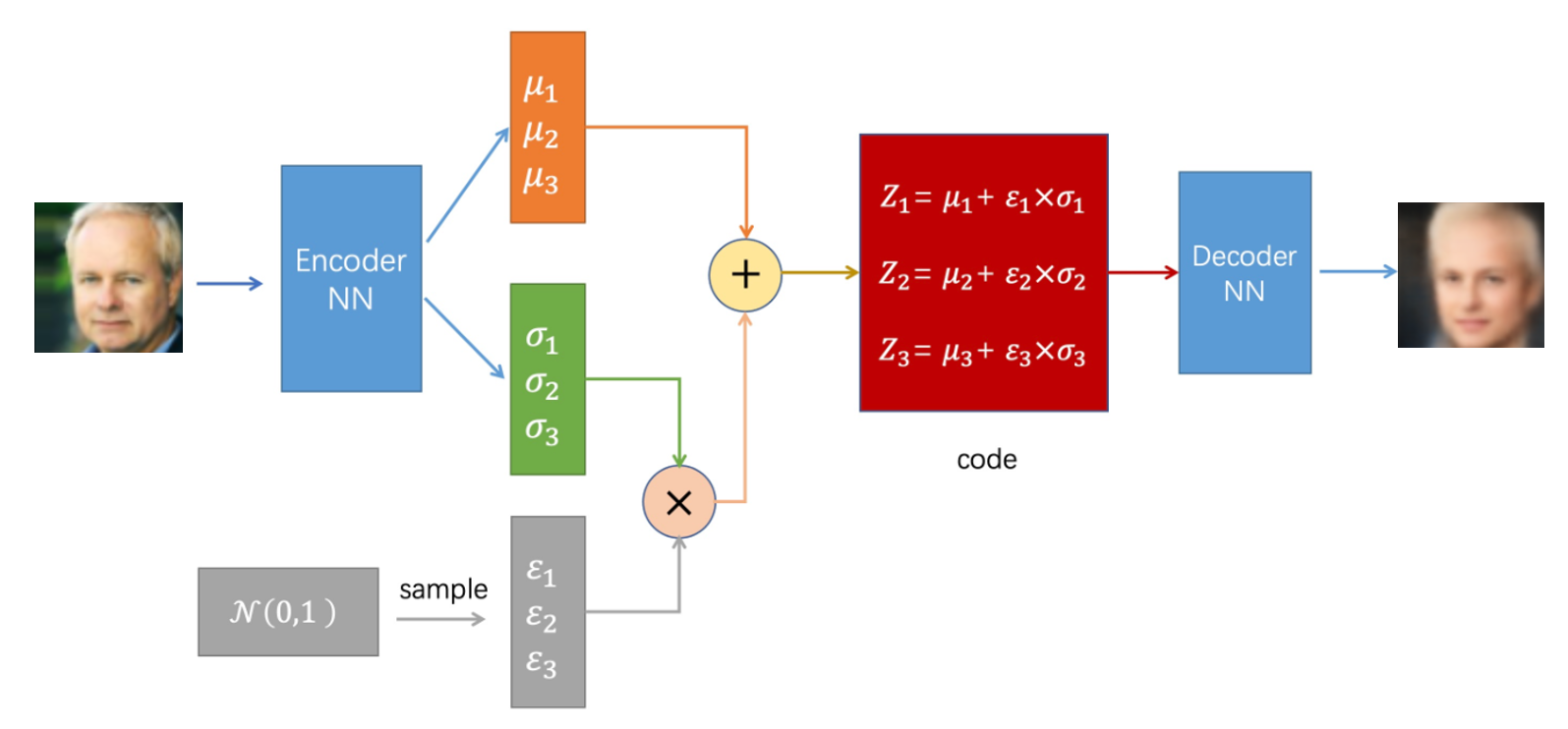• VAE预测阶段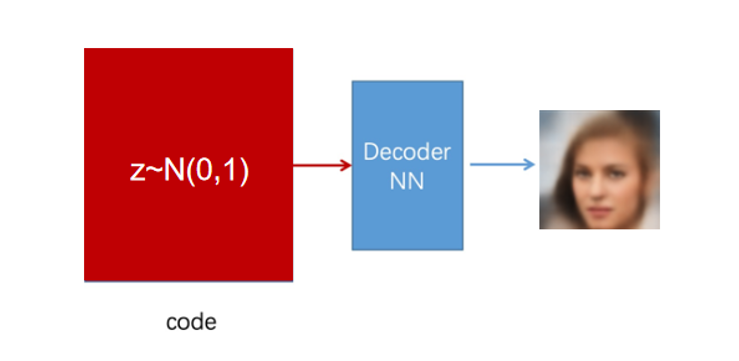VGAE是GCN在Variational Graph Auto-Encoders (VAE)的应用。Encoder用两个两层GCN分别得到N个均值和标准差，这两个GCN会共享第一层的参数，从而得到N个正态分布。Decoder仍然是向量点积。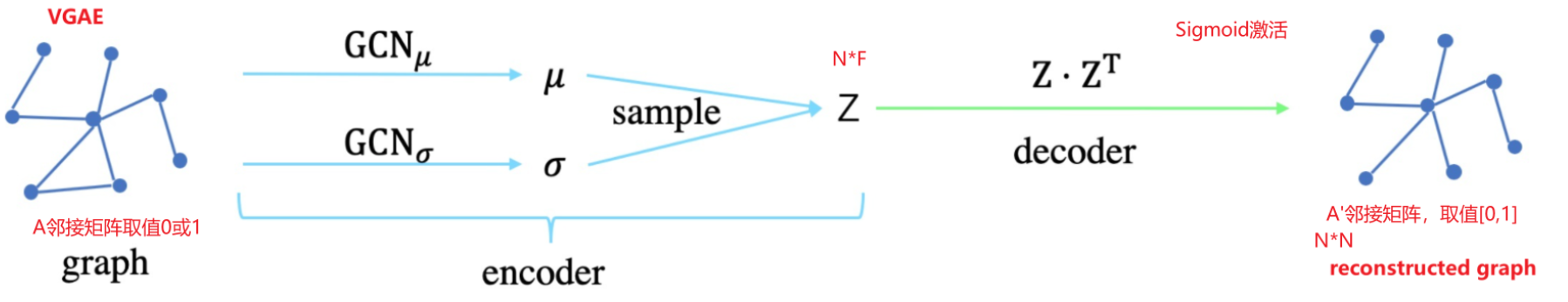VGAE是如何训练，它的loss函数是什么？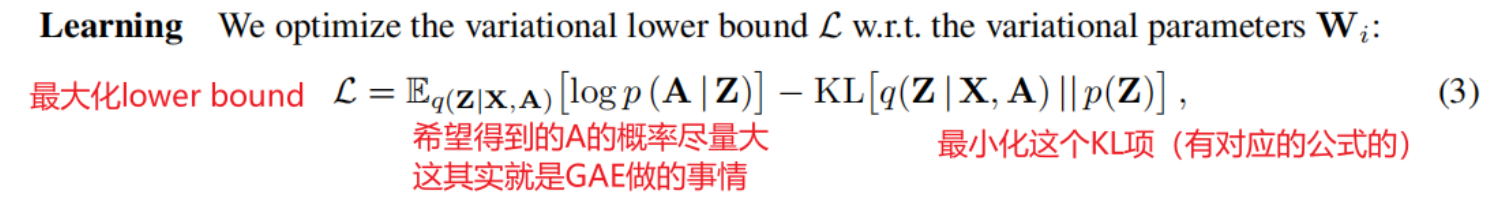We perform full-batch gradient descent and make use of the reparameterization trick for training.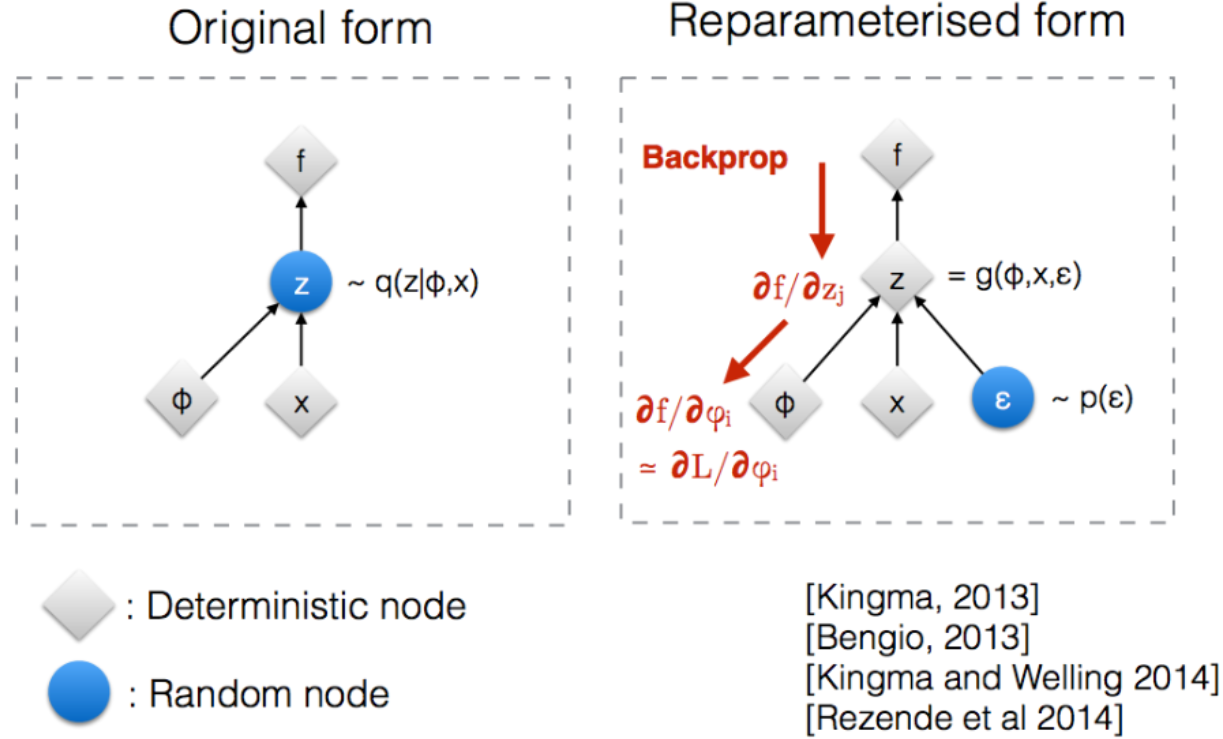# 四、实验结果

GAE和VGAE在解码的过程中都是在重构领接矩阵A，因此除了可以学习到节点的中间表示Z，它们还很适合链路预测的任务，最终得到的p(A|Z)可以用来预测A中任意未知元素的概率。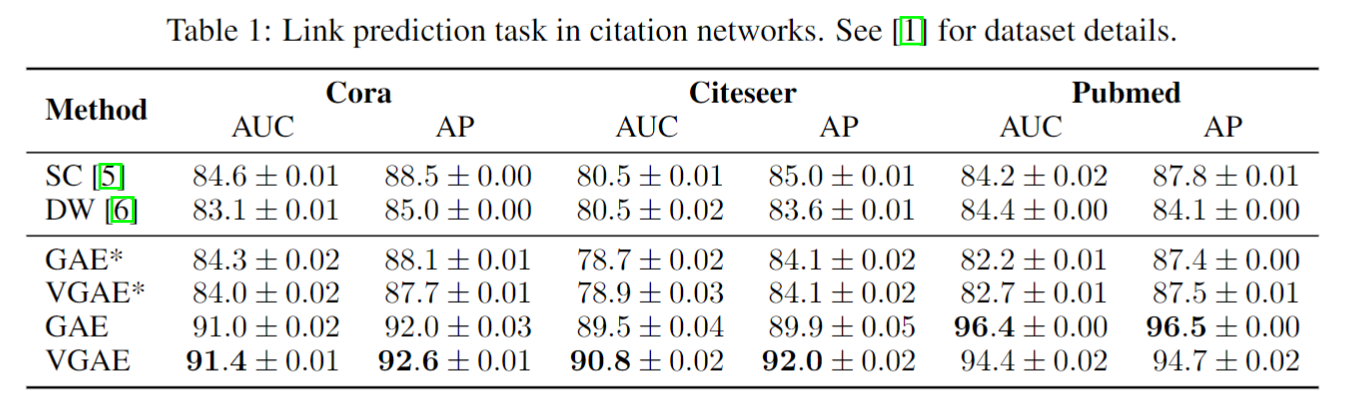# 六、参考资料

Author:
Reprint policy: All articles in this blog are used except for special statements CC BY 4.0 reprint polocy. If reproduced, please indicate source SHWEI !

Previous【GAN论文解读系列】NeurIPS 2016 InfoGAN 使用InfoGAN解耦出可解释的特征

2021-12-17
Next机器学习中极大似然估计MLE和最大后验估计MAP
(机器学习必知必会) 最大似然估计(MLE)、最大后验概率估计(MAP)这两个概念在机器学习和深度学习中经常碰到。现代机器学习的终极问题都会转化为解目标函数的优化问题，MLE和MAP是生成这个函数的很基本的思想，因此我们对二者的认知是非常
2021-11-24
TOC